Home  /  Products  /  Stata 14  /  IRT

#### IRT (item response theory) was introduced in Stata 14. See the latest version of irt. See all of Stata's irt features. See the new features in Stata 18.# IRT (item response theory)

## Highlights

• Binary response models
• One-parameter logistic (1PL)
• Two-parameter logistic (2PL)
• Three-parameter logistic (3PL)
• Ordinal response models
• Partial credit
• Rating scale
• Categorical response models
• Nominal response
• Hybrid models with differing response types
• Graphs
• Item characteristic curve
• Test characteristic curve
• Item information function
• Test information function
• Differential item functioning (DIF)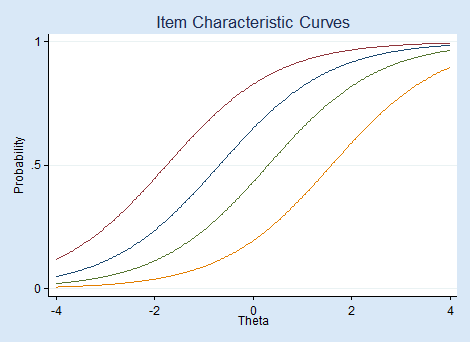×Many researchers study cognitive abilities, personality traits, attitudes, quality of life, patient satisfaction, and other attributes that cannot be measured directly. To quantify these types of latent traits, a researcher often develops an instrument—a questionnaire or test consisting of binary, ordinal, or categorical items—to determine individuals' levels of the trait.

Item response theory (IRT) models can be used to evaluate the relationships between the latent trait of interest and the items intended to measure the trait. With IRT, we can also determine how the instrument as a whole relates to the latent trait.

IRT is used when new instruments are developed, when analyzing and scoring data collected from these instruments, when comparing instruments that measure the same trait, and more.

For instance, when we develop a new instrument, we have a set of items that we believe to be good measurements of our latent trait. We can use IRT models to determine whether these items, or a subset, can be combined to form a good measurement tool. With IRT, we evaluate the amount of information each item provides. If some items do not provide much information, we may eliminate them. IRT models estimate the difficulty of each item. This tells us the level of the trait that is assessed by the item. We want items that provide information across the full continuum of the latent trait scale. We can also ask how much information an instrument, as a whole, provides for each level of the latent trait. If there are ranges of the latent trait for which little information is provided, we may add items to the test.

## Let's see it work

Suppose we have a test designed to assess mathematical ability based on eight questions that are scored 0 (incorrect) or 1 (correct). We fit a one-parameter logistic model, a model that estimates only the difficulty of each of our eight items, by typing

irt 1pl q1-q8


Even better, we can fit our model from the IRT Control Panel.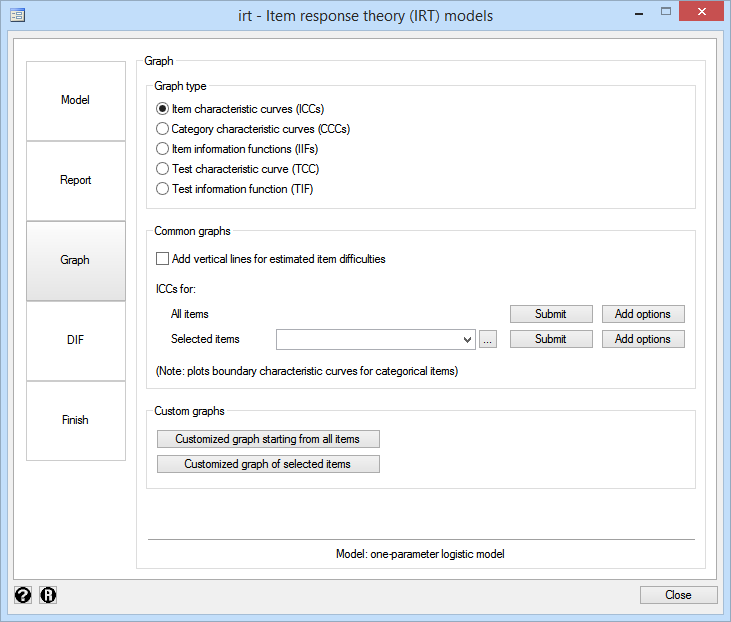Either way, here are the results.

. irt 1pl q1-q8

Fitting fixed-effects model:

Iteration 0:   log likelihood =   -3790.87
Iteration 1:   log likelihood =  -3786.285
Iteration 2:   log likelihood = -3786.2825
Iteration 3:   log likelihood = -3786.2825

Fitting full model:

Iteration 0:   log likelihood = -3681.3257
Iteration 1:   log likelihood = -3669.4837
Iteration 2:   log likelihood = -3669.4705
Iteration 3:   log likelihood = -3669.4705

One-parameter logistic model                   Number of obs     =        800
Log likelihood = -3669.4705

Coef.   Std. Err.      z    P>|z|     [95% Conf. Interval]

Discrim    .8926881   .0497697    17.94   0.000     .7951412     .990235

q1
Diff   -.6826695   .1000551    -6.82   0.000    -.8787739    -.486565

q2
Diff   -.1177849   .0930488    -1.27   0.206    -.3001572    .0645875

q3
Diff   -1.754276   .1356766   -12.93   0.000    -2.020197   -1.488355

q4
Diff    .3101872   .0943281     3.29   0.001     .1253074    .4950669

q5
Diff    1.595213   .1288328    12.38   0.000     1.342705    1.847721

q6
Diff    .6694488   .0997334     6.71   0.000     .4739748    .8649227

q7
Diff    1.279229   .1167531    10.96   0.000     1.050397    1.508061

q8
Diff   -2.328184   .1640633   -14.19   0.000    -2.649742   -2.006625



Coefficients labeled "Diff" report difficulty. Based on this model, question 8 is the easiest with a coefficient of −2.328. Question 5 is the most difficult with a coefficient of 1.595.

We have only eight questions in our example. If we had 50 questions, it would not be as easy to spot those that correspond to a particular difficulty level. We can use estat report to sort the questions by difficulty.

. estat report, sort(b) byparm

One-parameter logistic model                   Number of obs     =        800
Log likelihood = -3669.4705

Coef.   Std. Err.      z    P>|z|     [95% Conf. Interval]

Discrim    .8926881   .0497697    17.94   0.000     .7951412     .990235

Diff
q8   -2.328184   .1640633   -14.19   0.000    -2.649742   -2.006625
q3   -1.754276   .1356766   -12.93   0.000    -2.020197   -1.488355
q1   -.6826695   .1000551    -6.82   0.000    -.8787739    -.486565
q2   -.1177849   .0930488    -1.27   0.206    -.3001572    .0645875
q4    .3101872   .0943281     3.29   0.001     .1253074    .4950669
q6    .6694488   .0997334     6.71   0.000     .4739748    .8649227
q7    1.279229   .1167531    10.96   0.000     1.050397    1.508061
q5    1.595213   .1288328    12.38   0.000     1.342705    1.847721



We can visualize the relationship between questions and mathematical ability—between items and latent trait—by graphing the item characteristic curves (ICCs) using irtgraph icc.We made the easiest question blue and the hardest, red. The probability of succeeding on the easiest question is higher than the probability of succeeding on all other questions. Because we fit a 1PL model, this is true at every level of ability.

irtgraph tif graphs the test information function.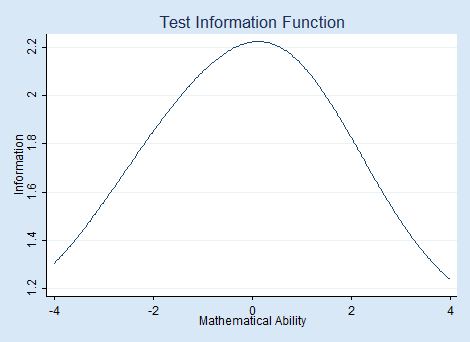The hump in the middle shows that this test provides the most information for average mathematical ability levels.

When we have binary items, we can fit a 1PL, 2PL, or 3PL model. The irt 2pl command fits a 2PL model and allows items to have different difficulties and different abilities to discriminate between high and low levels of the latent trait. Visually, differing discriminations means that the slopes of our ICC curves differ across items. The irt 3pl command extends the 2PL model to allow for the possibility of guessing correct answers.

## Let's see it work with ordinal items

IRT models can be fit to ordinal and categorical items, too. Here we have a new test, also with eight questions. Individuals are expected to show their work as they solve each problem. Responses are scored as 0 (incorrect), 1 (partially correct), or 2 (correct).

With ordinal data, we could fit a graded response model, a partial credit model, or a rating scale model. These models make different assumptions about how the ordered scores relate to the latent trait. Here we fit a graded response model by typing

irt grm q1-q8


The results are

. irt grm q1-q8

Fitting fixed-effects model:

Iteration 0:   log likelihood = -20869.947
Iteration 1:   log likelihood = -20869.947

Fitting full model:

Iteration 0:   log likelihood =  -19891.74
Iteration 1:   log likelihood = -19644.197
Iteration 2:   log likelihood = -19634.227
Iteration 3:   log likelihood = -19634.173
Iteration 4:   log likelihood = -19634.173

Graded response model                          Number of obs     =      2,941
Log likelihood = -19634.173

Coef.   Std. Err.      z    P>|z|     [95% Conf. Interval]

q1
Discrim     1.75666   .1081946    16.24   0.000     1.544603    1.968718
Diff
>=1    -2.138871   .0879781   -24.31   0.000    -2.311305   -1.966437
=2    -1.238469   .0530588   -23.34   0.000    -1.342462   -1.134476

q2
Discrim    1.575855   .0888881    17.73   0.000     1.401637    1.750072
Diff
>=1    -1.345774   .0596825   -22.55   0.000     -1.46275   -1.228798
=2    -.5571402   .0383507   -14.53   0.000    -.6323062   -.4819741

q3
Discrim    1.100984   .0626802    17.57   0.000     .9781335    1.223835
Diff
>=1    -1.604283   .0847658   -18.93   0.000    -1.770421   -1.438145
=2     .1580019    .041744     3.79   0.000     .0761851    .2398186

q4
Discrim    .9245333   .0549971    16.81   0.000     .8167411    1.032326
Diff
>=1    -.7752653   .0616916   -12.57   0.000    -.8961786   -.6543519
=2     1.227147    .076343    16.07   0.000     1.077518    1.376776

q5
Discrim    1.528995   .0896173    17.06   0.000     1.353349    1.704642
Diff
>=1     .0365143   .0342353     1.07   0.286    -.0305857    .1036143
=2     .3670961   .0366753    10.01   0.000     .2952138    .4389784

q6
Discrim    .6986686   .0516767    13.52   0.000     .5973842     .799953
Diff
>=1    -.3764114   .0636946    -5.91   0.000    -.5012505   -.2515723
=2     4.226257   .2991581    14.13   0.000     3.639917    4.812596

q7
Discrim    1.430949   .0874188    16.37   0.000     1.259611    1.602286
Diff
>=1     .6332313   .0418799    15.12   0.000     .5511482    .7153143
=2     1.242491   .0612475    20.29   0.000     1.122448    1.362534

q8
Discrim    .9449605   .0647013    14.60   0.000     .8181483    1.071773
Diff
>=1     1.041434   .0704925    14.77   0.000     .9032708    1.179596
=2      2.72978   .1638681    16.66   0.000     2.408605    3.050956



One way to evaluate how an individual item, say, q3, relates to mathematical ability is to look at the category characteristic curves produced by irtgraph icc.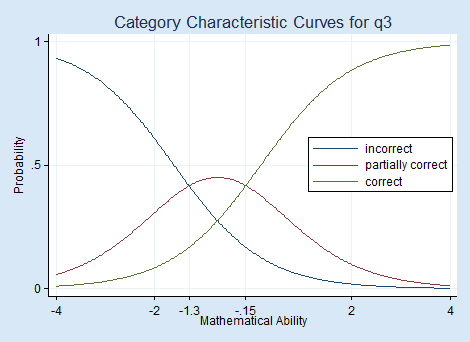Respondents with mathematical ability levels below −1.3 are most likely to answer q3 with a completely incorrect answer, those with levels between −1.3 and −0.2 are most likely to give a partially correct answer, and those with ability levels above −0.15 are most likely to give a completely correct answer.

From the test characteristic curve produced by irtgraph tcc, we see how the expected total test score relates to mathematical ability levels.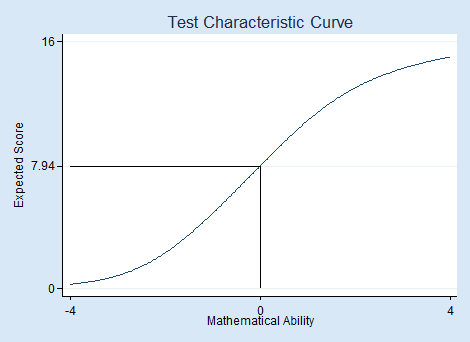Out of a possible 16 points on the test, a person with above-average mathematical ability (above 0) is expected to score above 7.94 or, because all scores are integers, above 7.

## Not interested in standardized testing?

IRT models can be used to measure many types of latent traits. For example,

• attitudes
• personality traits
• health outcomes
• quality of life

Use IRT for analyzing any unobservable characteristic for which binary or categorical measurements are observed.

## Tell me more

Stata's new IRT features are documented in their own new 193-page manual. You can read more about IRT and more about Stata's new IRT features and see several worked examples in Stata Item Response Theory Reference Manual.